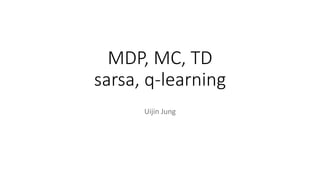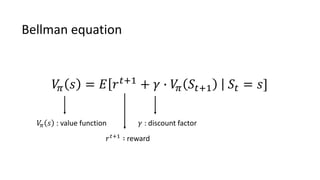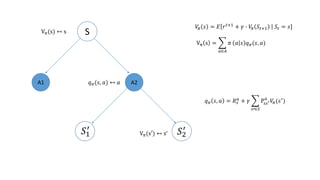Diese Präsentation wurde erfolgreich gemeldet.

# Reinforcement Learning basics part1

Anzeige
Anzeige
Anzeige
Anzeige
Anzeige
Anzeige
Anzeige
Anzeige
Anzeige
Anzeige
Anzeige×

1 von 20 Anzeige

# Reinforcement Learning basics part1

It described about MDP, Monte-Carlo, Time-Difference, sarsa, and q-learning method, and used for Reinforcement Learning study group's lecture, where is belonged to Korea Artificial Intelligence Laboratory.

It described about MDP, Monte-Carlo, Time-Difference, sarsa, and q-learning method, and used for Reinforcement Learning study group's lecture, where is belonged to Korea Artificial Intelligence Laboratory.

Anzeige
Anzeige

### Reinforcement Learning basics part1

1. 1. MDP, MC, TD sarsa, q-learning Uijin Jung
2. 2. 𝑉𝜋 𝑠 = 𝐸 𝑟 𝑡+1 + 𝛾 ∙ 𝑉𝜋 𝑆𝑡+1 𝑆𝑡 = 𝑠] Bellman equation 𝑉𝜋 𝑠 : value function 𝑟 𝑡+1 ∶ reward 𝛾 : discount factor
3. 3. S A1 A2 Vπ(s) ↤ s 𝑞 𝜋(𝑠, 𝑎) ↤ 𝑎 Vπ s = 𝑎∈𝐴 𝜋 𝑎 𝑠 𝑞 𝜋(𝑠, 𝑎) Vπ(s′) ↤ s’ 𝑞 𝜋 𝑠, 𝑎 = 𝑅 𝑠 𝑎 + 𝛾 𝑠′∈𝑆 Ρ𝑠𝑠′ 𝑎 𝑉𝜋(𝑠′ ) 𝑆1 ′ 𝑆2 ′ 𝑉𝜋 𝑠 = 𝐸 𝑟 𝑡+1 + 𝛾 ∙ 𝑉𝜋 𝑆𝑡+1 𝑆𝑡 = 𝑠]
4. 4. Vπ s = 𝑎∈𝐴 𝜋 𝑎 𝑠 𝑞 𝜋(𝑠, 𝑎) 𝑞 𝜋 𝑠, 𝑎 = 𝑅 𝑠 𝑎 + 𝛾 𝑠′∈𝑆 Ρ𝑠𝑠′ 𝑎 𝑉𝜋(𝑠′ ) Vπ s = 𝑎∈𝐴 𝜋 𝑎 𝑠 (𝑅 𝑠 𝑎 + 𝛾 𝑠′∈𝑆 Ρ𝑠𝑠′ 𝑎 𝑉𝜋(𝑠′ ))
5. 5. Limitation of Bellman equation • We must know MDP model perfectly.
6. 6. Monte-Carlo • Learn from the episodes • Action selected by policy-> end of episode-> calculate value function based on the episode • If you iterate an episode several time, calculate value function by averaging each rewards took from the state 𝑠𝑡.
7. 7. = 1 𝑡 𝐺𝑡 + 𝑗=1 𝑡−1 𝐺𝑗 𝑗=1 𝑡−1 𝐺𝑗 = (𝑡 − 1)𝑉𝑡−1 = 1 𝑡 𝐺𝑡 + (𝑡 − 1)𝑉𝑡−1 𝑉𝑡 = 1 𝑡 𝑗=1 𝑡 𝐺𝑗 𝑉𝑡−1 = 1 𝑡 − 1 𝑗=1 𝑡−1 𝐺𝑗 (𝑡 − 1)𝑉𝑡−1= 𝑗=1 𝑡−1 𝐺𝑗
8. 8. 𝑉𝑡 = 1 𝑡 𝐺𝑡 + (𝑡 − 1)𝑉𝑡−1 = 1 𝑡 𝐺𝑡 + 𝑉𝑡−1 − 1 𝑡 ∙ 𝑉𝑡−1 = 𝑉𝑡−1 + 1 𝑡 𝐺𝑡 − 𝑉𝑡−1 𝑉𝑡−1 + 1 𝑡 𝐺𝑡 − 1 𝑡 ∙ 𝑉𝑡−1 = 𝑉𝑡−1 + 𝑎 𝐺𝑡 − 𝑉𝑡−1
9. 9. 𝑉𝑡 = 𝑉𝑡−1 + 𝑎 𝐺𝑡 − 𝑉𝑡−1 Reward predicted by previous value function Actual reward Previous value function Value function 𝑉𝜋 𝑠𝑡 ← 𝑉𝜋(𝑠𝑡) + 𝑎 𝐺𝑡 − 𝑉(𝑠𝑡) This is how we update value function Using Monte-Carlo method
10. 10. Limitations of Monte-Carlo • Episode must end before we update the value function. • It is difficult to train if the environment is endless or if the time to finish is too long.
11. 11. Time difference • Let's change the Monte-Carlo method to a real time processing method. 𝐺𝑡 = 𝑅𝑡+1 + 𝛾𝐺𝑡+1 𝑅𝑡+1 + 𝛾𝑉 (𝑆𝑡+1) 𝑉𝑡 = 𝑉𝑡 + 𝑎 𝑅𝑡+1 + 𝛾𝑉 (𝑆𝑡+1) − 𝑉𝑡 𝑉𝜋 𝑠𝑡 ← 𝑉𝜋(𝑠𝑡) + 𝑎 𝐺𝑡 − 𝑉(𝑠𝑡)
12. 12. Time difference • advantage • You can update the value function in real time. • disadvantage • The reference 𝐺𝑡 is not true, it is derived from the expected value : (we call this phenomenon, bootstrap)
13. 13. Sarsa • Algorithm to find optimal q value through TD method 𝑄 𝑆, 𝐴 ← 𝑄 𝑆, 𝐴 + 𝛼(𝑅 + 𝛾𝑄 𝑆′, 𝐴′ − 𝑄 𝑆, 𝐴 ) State, Action, Reward, next State, next Action
14. 14. Sarsa pseudo code (https://stackoverflow.com/questions/32846262/q-learning-vs-sarsa-with-greedy-select)
15. 15. Me exploration predict Sarsa -1 +1 • On-Policy • There is a possibility of being biased.
16. 16. Q-learaning • Algorithm to find optimal q value through TD method using off-policy.
17. 17. Sarsa Q-learning 𝑄 𝑠, 𝑎 ← 𝑄 𝑠, 𝑎 + 𝛼(𝑅 + 𝛾𝑄 𝑠′, 𝑎′ − 𝑄 𝑠, 𝑎 ) 𝑄 𝑠, 𝑎 ← 𝑄(𝑠, 𝑎) + 𝛼(𝑅 + 𝛾 ∙ 𝑚𝑎𝑥 𝑎′ 𝑄 𝑠′ , 𝑎′ − 𝑄 𝑠, 𝑎 )
18. 18. Q-learning pseudo code (https://stackoverflow.com/questions/32846262/q-learning-vs-sarsa-with-greedy-select)
19. 19. Me exploration predict Q-learning -1 +1 v
20. 20. The end